#### Risk Disclosure

Futures, foreign currency and options trading contains substantial risk and is not for every investor. An investor could potentially lose all or more than the initial investment. Risk capital is money that can be lost without jeopardizing ones financial security or lifestyle. Only risk capital should be used for trading and only those with sufficient risk capital should consider trading. Past performance is not necessarily indicative of future results. View full risk disclosure.

## Crossover Solver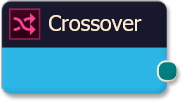The Crossover solver generates a favorable output (signal) when Indicator A crossesIndicator B.  The signal is given in the direction of the Indicator A.  I.E. if Indicator A crosses aboveIndicator B then a Long signal is given.

# BloodHound   v2

This section is specific to BloodHound v2.  Scroll down for BloodHound v1.

## Properties tab

This tab contains the essential settings for all nodes.

### Modifiers

Please note:   The buttons only modify the individual instance of the selected node(s) on a Logic Board.   Thus, the buttons are only visible when a node is selected on the Logic Board.  The buttons are not available when a solver is selected in the Solvers panel, because the original solver's output can not be globally modified.
This behavior is consistent with adding a function node afterwards, so that the original solver's output remains unmodified elsewhere in the system.  It is similar to having an SMA(50) on several charts.  Changing the plot color on one chart does not modify the plot color on the other charts.

### Global Properties

These setting apply to all instances of the selected solver.  Changing a setting in one of the instances changes the setting in all instances.  If you only want to change one of the solvers, but not the others, you must make a copy of the solver.  The copy will be a different solver, and a number will be added at the end of the name to differentiate it from the original.

### Behavior

This option determines which outputs are calculated.  Effectively turning off either the Long or Short output.

#### Input A

This is the first input data to be evaluated against Input B.  Use the Type drop-down menu to select the data type.
The menu will change based on which data type is selected.  Click on Type to see the various data types available and the corresponding documentation.

The Input section determines the data type to be evaluated.  Use the 'Type' menu to select the data type.  See below for detailed information on each data type.

##### Type:   Price

This option allows price data to be used in the solver.  The price data can be shaped/manipulated by the various settings before it is used by the solver.

Custom Prices
SharkIndicators has custom system development prices that are very useful in reducing the number of solvers, and simplifying system logic, when evaluating the open and close (body) prices.
Body Top:  Regardless of the bar direction, this returns the upper price of the candlestick body.  Either the close or open price.  Whichever is on top.  e.g.  For an up bar, the Body Top equals the close price.
Body Median:    This returns the median price of the candlestick body.  The formula is (Close + Open) ÷ 2.
Body Bottom:    Regardless of the bar direction, this returns the lower price of the candlestick body.  Either the close or open price.  Whichever is the bottom.  e.g.  For an up bar, the Body Bottom equals the open price.

##### Type:   Volume

Volume will feed the current bar volume into the solver.
To evaluate the volume from a few bars back, use Indicator Value.  Then select the VOL indicator, and use the Displacement setting.

##### Type:   Swing Point Prices

Swing Point Prices uses the Swing Highs & Lows indicator plots for input price data.  This is useful when you need to access the lowest low or highest high prices from the latest swing point.
You can add the bundled indicator Swing Highs & Lows to the chart to help you visualize the price data the solver is using.

#### Input B

This is the second input data to be evaluated against Input A.  For  documentation scroll up to section Input A.
Use the Type drop-down menu to select the data type.  The menu will change based on which data type is selected.

## Options tab

This tab contains extra secondary settings common to all nodes.

### Weight

This section is used to modify the node's final output value.  It is only useful for designing fuzzy logic systems, or a fuzzy logic section of a system.
Please note:   The Weight controls only modify the individual instance of the selected node(s) on a Logic Board.   Thus, the Weight controls are only visible when a node is selected on the Logic Board.  The controls are not available when a solver is selected in the Solvers panel, because the original solver's output can not be globally modified.
This behavior is different than BloodHound 1.0.  In BH 1.0, the Weight controls modified the nodes output globally (every instance).  This change in BH 2.0 adds more system design granularity.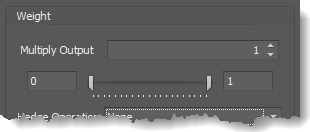Multiply Output:   This takes the internal values (the Long and Short values determined via the Properties tab » Output Rules section) and multiplies them by this value.  Note: the final output will not exceed a value of 1, as described in the Slider control below.

Slider control:  The slider constrains the final output to a value of 0 to 1.  The left side of the slider sets a minimum value that is output regardless if the solver condition is true or not.  The right side sets a maximum value that is output.  The output is capped.
e.g.  Three indicator conditions are being checked, and thus three solvers are created.  Only two out of the three indicator conditions are needed.  An Additive logic node is used to add the solver's outputs together.  Just two out of the three solvers need to add up to a value of 1.  Therefore, the right slider (max output value) for all three solvers is set to 0.5.  When two indicator/solver conditions are found, thus the outputs = 0.5, then the calculation, in the Additive node, is 0.5 + 0.5 + 0 = 1.  A value of 1 means the two out of three condition is true.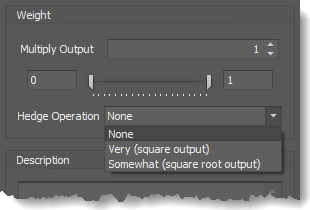Hedge Operation: This applies a mathematical formula to the internal value.

None:  No modification is applied.
Very (square output):  A squaring formula is applied.  Output  =  value .
Somewhat (square root output):  A square root formula is applied.  Output  =  √value .

### Description

This text area provides a place to write a full description of what the node is doing, used for, or what ever you want.
Note:   The Description is global to all instances of the node.  It is not applied individually to each instance as the Weight controls are.

## Help tab

This tab displays the documentation page (from this web site) of the selected node.
Please note:  NinjaTrader v8.0.26.0 or newer is required for the built in web viewer to work, and thus the documentation to be displayed.

### Video Tutorial

This video is from our weekly Workshop on June 12th, 2015.
For more benefit please watch in full screen mode, as this video is recorded in HD.
This video shows BloodHound v1, however the settings and usage are the same.

### Examples

The screenshots below show BloodHound v1, however the settings and usage are still the same.

#### Example 1: The Basics

This demonstrates the basic function of Indicator Crossover solver with the use of a 10 SMA & 30 EMA.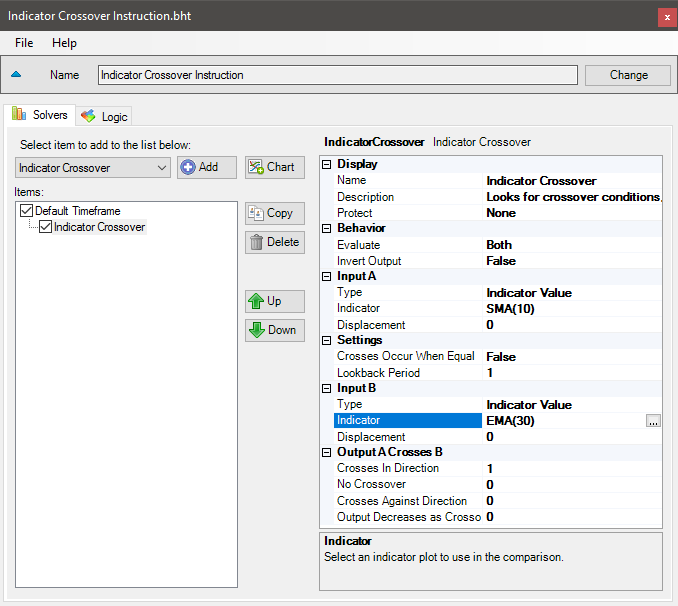• Add the Indicator Crossover solver
• Set Indicator A to SMA 10
• Set Indicator B to EMA 30

The chart below shows a Long signal when the SMA 10 crosses above the EMA 30, and a Short signal when the SMA 10 crosses below the EMA 30.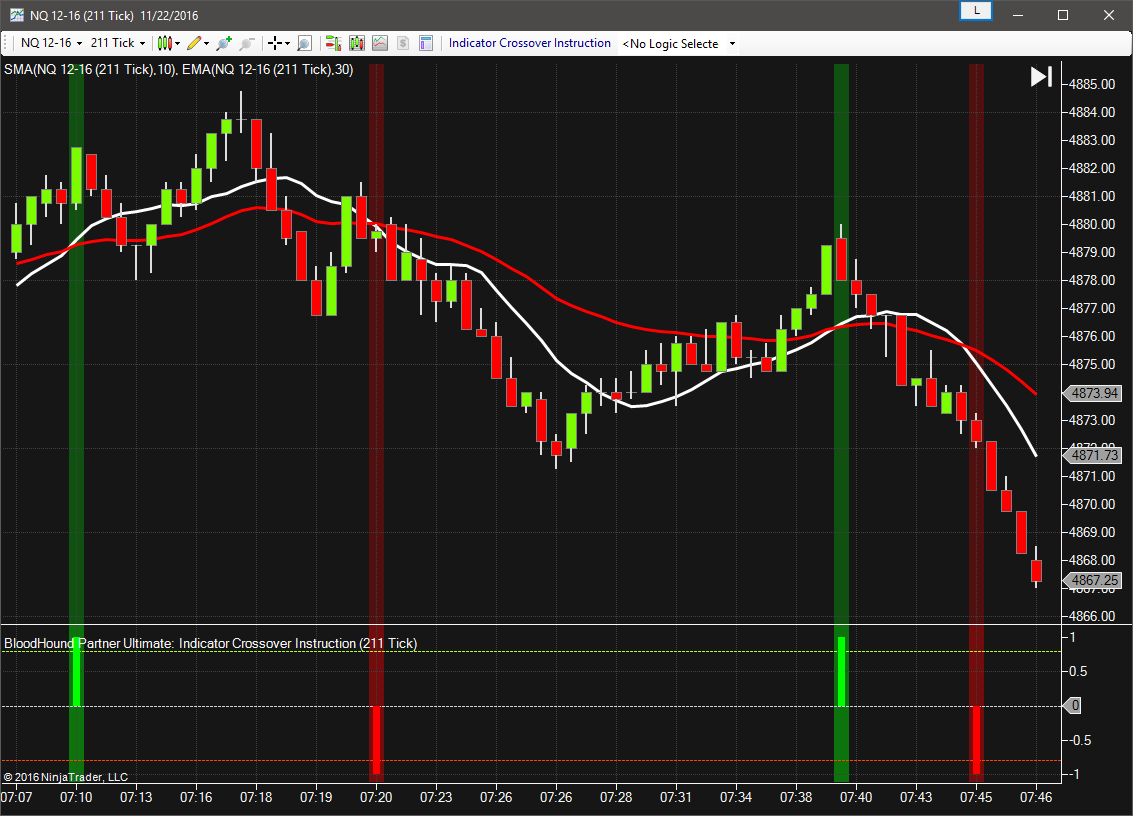#### Example 2: Threshold Filter

This example uses the Stochastic K crossing the D.  Longs are permitted when the Stochastic D is below 45, and Shorts are permitted when the Stochastic D is above 55.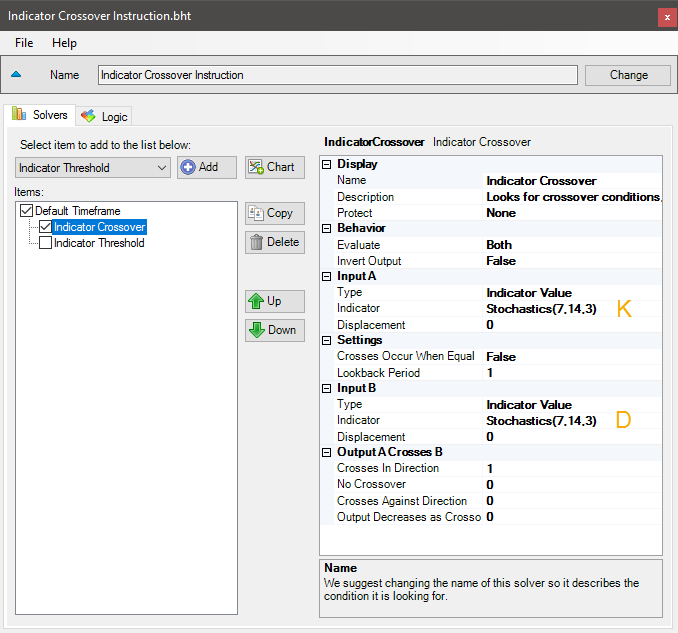• Add the Indicator Crossover solver
• Set Indicator A to Stochastic K
• Set Indicator B to Stochastic D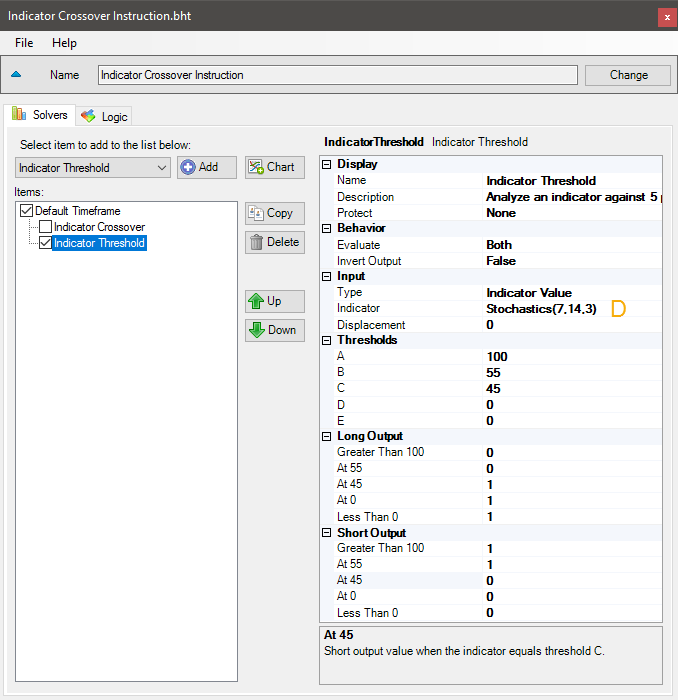• Add the Indicator Threshold solver (the permissive)
• Set Indicator to Stochastic D
• Set Threshold A to 100, B to 55, C to 45, D to 0
• Set Long Output At C & At D to 1
• Set Short Output At A & At B to 1

This chart shows the Crossover solver only.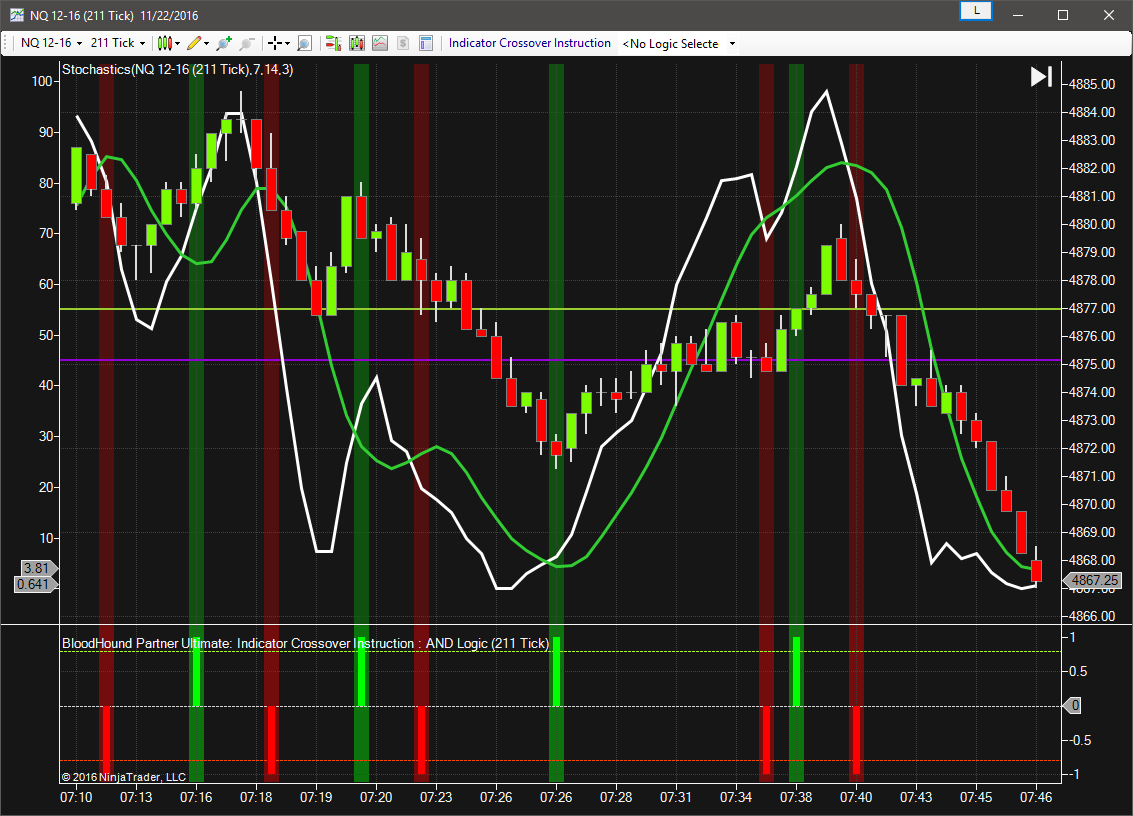This chart shows the Threshold solver only.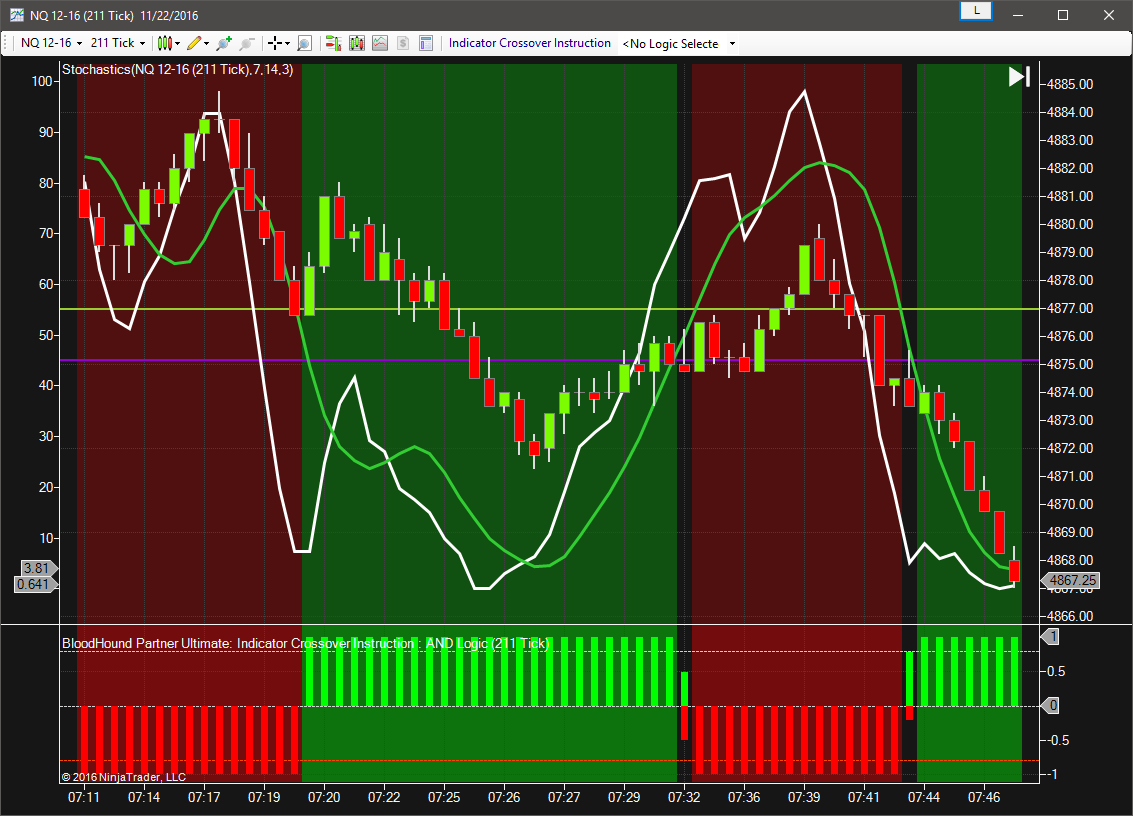The chart below has both solvers enabled showing the signals as described above.  Notice in this simple 2 solver system that the Threshold solver output is seen showing the permissive for Longs or Shorts.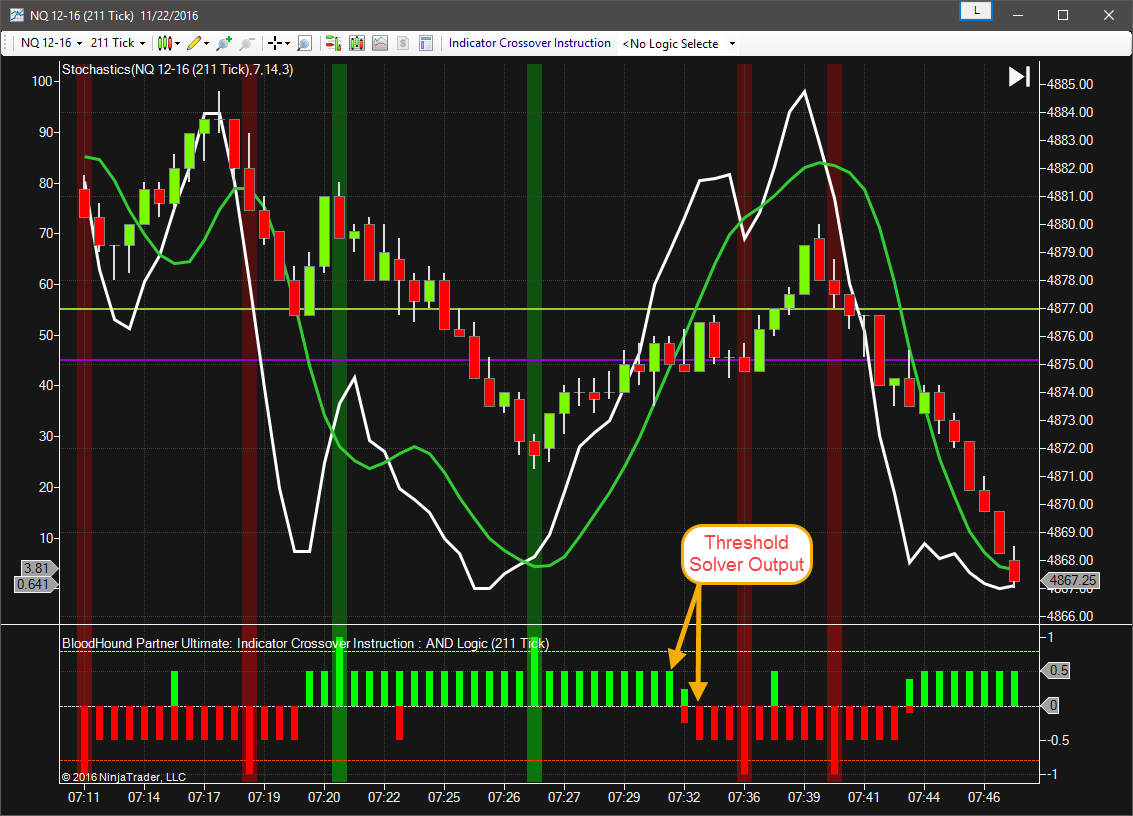#### Example 3: AND Logic Node

Now lets use a AND logic node to observe how the output values change.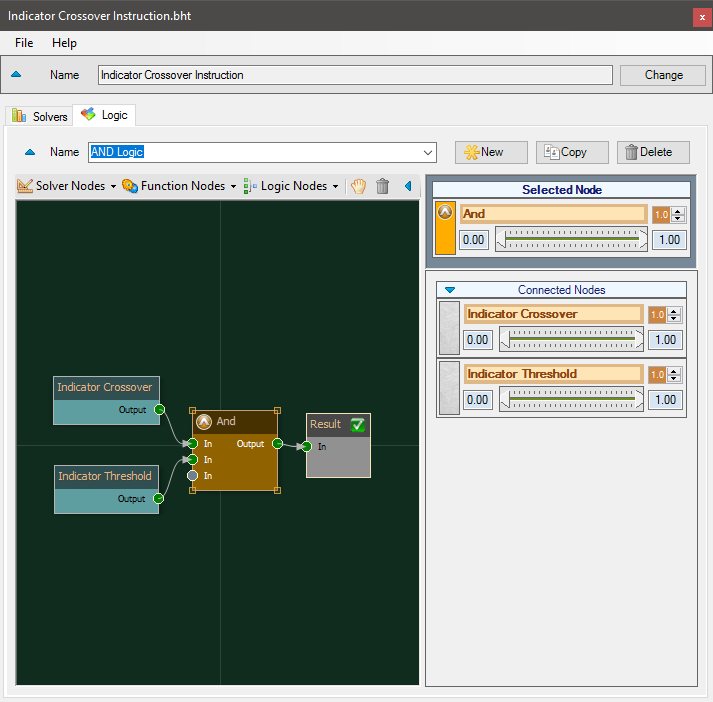• Switch to the Logic tab, and click New
• Add a AND logic node
• Connect the leaders as shown

Here we see the AND node gives an output only when both solvers have an output in the same direction.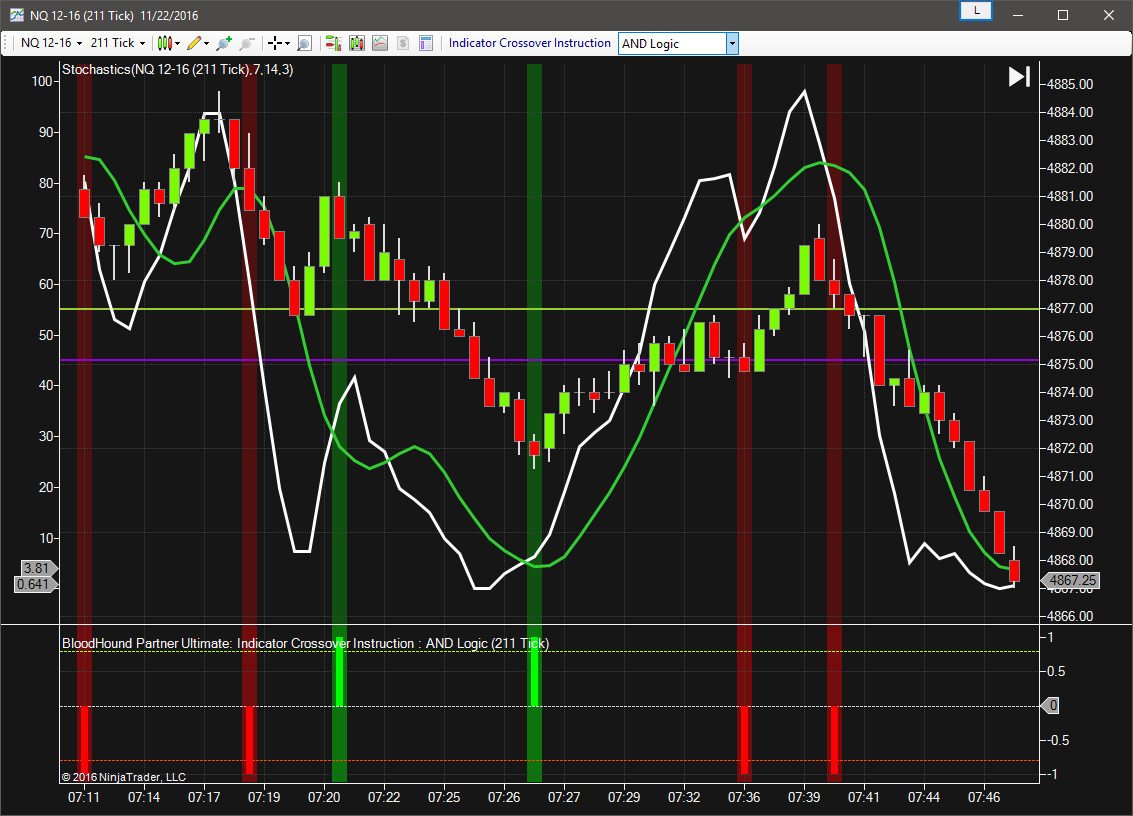#### Risk Disclosure

Futures, foreign currency and options trading contains substantial risk and is not for every investor. An investor could potentially lose all or more than the initial investment. Risk capital is money that can be lost without jeopardizing ones financial security or lifestyle. Only risk capital should be used for trading and only those with sufficient risk capital should consider trading. Past performance is not necessarily indicative of future results. View full risk disclosure.

# BloodHound   v1

This section is specific to BloodHound v1.  Scroll up for BloodHound v2.

# Indicator Crossover SolverThe Crossover solver generates a favorable output (signal) when Indicator A crossesIndicator B.  The signal is given in the direction of the Indicator A.  I.E. if Indicator A crosses aboveIndicator B then a Long signal is given.

### Video Tutorial

This video is from our weekly Workshop on June 12th, 2015.
For more benefit please watch in full screen mode, as this video is recorded in HD.

### Examples

#### Example 1: The Basics

This demonstrates the basic function of Indicator Crossover solver with the use of a 10 SMA & 30 EMA.• Add the Indicator Crossover solver
• Set Indicator A to SMA 10
• Set Indicator B to EMA 30

The chart below shows a Long signal when the SMA 10 crosses above the EMA 30, and a Short signal when the SMA 10 crosses below the EMA 30.#### Example 2: Threshold Filter

This example uses the Stochastic K crossing the D.  Longs are permitted when the Stochastic D is below 45, and Shorts are permitted when the Stochastic D is above 55.• Add the Indicator Crossover solver
• Set Indicator A to Stochastic K
• Set Indicator B to Stochastic D• Add the Indicator Threshold solver (the permissive)
• Set Indicator to Stochastic D
• Set Threshold A to 100, B to 55, C to 45, D to 0
• Set Long Output At C & At D to 1
• Set Short Output At A & At B to 1

This chart shows the Crossover solver only.This chart shows the Threshold solver only.The chart below has both solvers enabled showing the signals as described above.  Notice in this simple 2 solver system that the Threshold solver output is seen showing the permissive for Longs or Shorts.#### Example 3: AND Logic Node

Now lets use a AND logic node to observe how the output values change.• Switch to the Logic tab, and click New
• Add a AND logic node
• Connect the leaders as shown

Here we see the AND node gives an output only when both solvers have an output in the same direction.#### Risk Disclosure

Futures, foreign currency and options trading contains substantial risk and is not for every investor. An investor could potentially lose all or more than the initial investment. Risk capital is money that can be lost without jeopardizing ones financial security or lifestyle. Only risk capital should be used for trading and only those with sufficient risk capital should consider trading. Past performance is not necessarily indicative of future results. View full risk disclosure.

## Indicator Crossover SolverThe Crossover solver generates a favorable output (signal) when Indicator A crossesIndicator B.  The signal is given in the direction of the Indicator A.  I.E. if Indicator A crosses aboveIndicator B then a Long signal is given.

### Video Tutorial

This video is from our weekly Workshop on June 12th, 2015.
For more benefit please watch in full screen mode, as this video is recorded in HD.

### Examples

#### Example 1: The Basics

This demonstrates the basic function of Indicator Crossover solver with the use of a 10 SMA & 30 EMA.• Add the Indicator Crossover solver
• Set Indicator A to SMA 10
• Set Indicator B to EMA 30

The chart below shows a Long signal when the SMA 10 crosses above the EMA 30, and a Short signal when the SMA 10 crosses below the EMA 30.#### Example 2: Threshold Filter

This example uses the Stochastic K crossing the D.  Longs are permitted when the Stochastic D is below 45, and Shorts are permitted when the Stochastic D is above 55.• Add the Indicator Crossover solver
• Set Indicator A to Stochastic K
• Set Indicator B to Stochastic D• Add the Indicator Threshold solver (the permissive)
• Set Indicator to Stochastic D
• Set Threshold A to 100, B to 55, C to 45, D to 0
• Set Long Output At C & At D to 1
• Set Short Output At A & At B to 1

This chart shows the Crossover solver only.This chart shows the Threshold solver only.The chart below has both solvers enabled showing the signals as described above.  Notice in this simple 2 solver system that the Threshold solver output is seen showing the permissive for Longs or Shorts.#### Example 3: AND Logic Node

Now lets use a AND logic node to observe how the output values change.• Switch to the Logic tab, and click New
• Add a AND logic node
• Connect the leaders as shown

Here we see the AND node gives an output only when both solvers have an output in the same direction.>